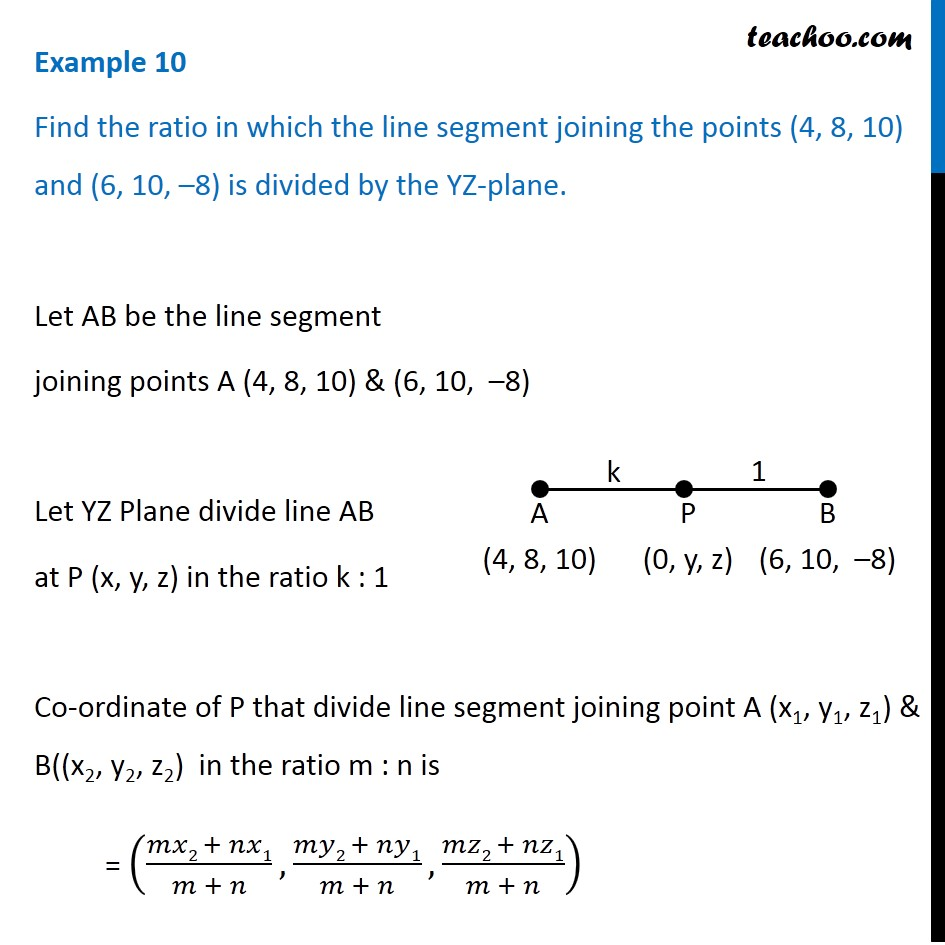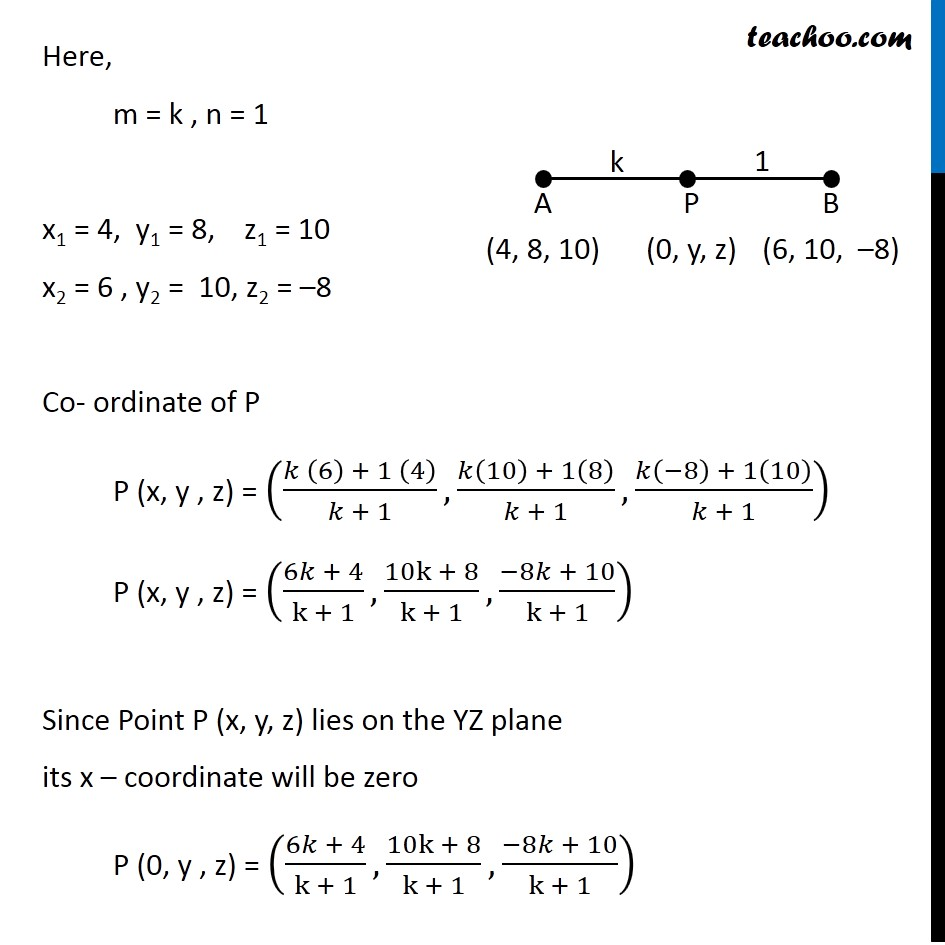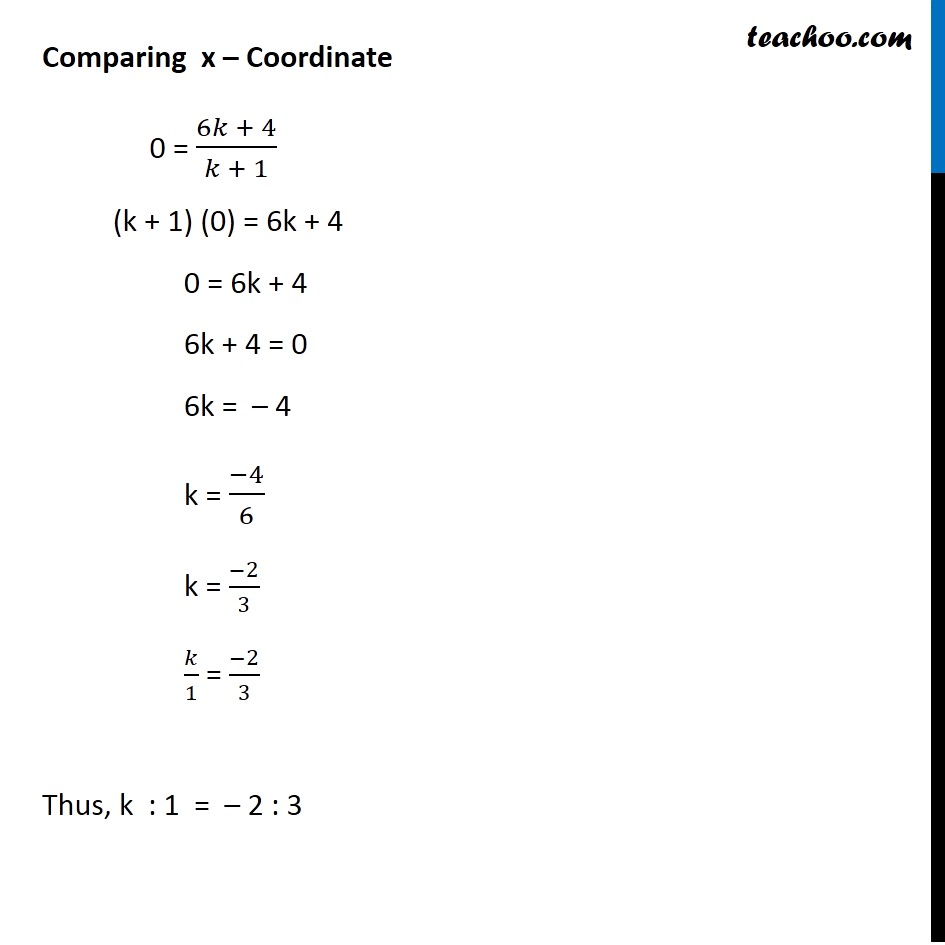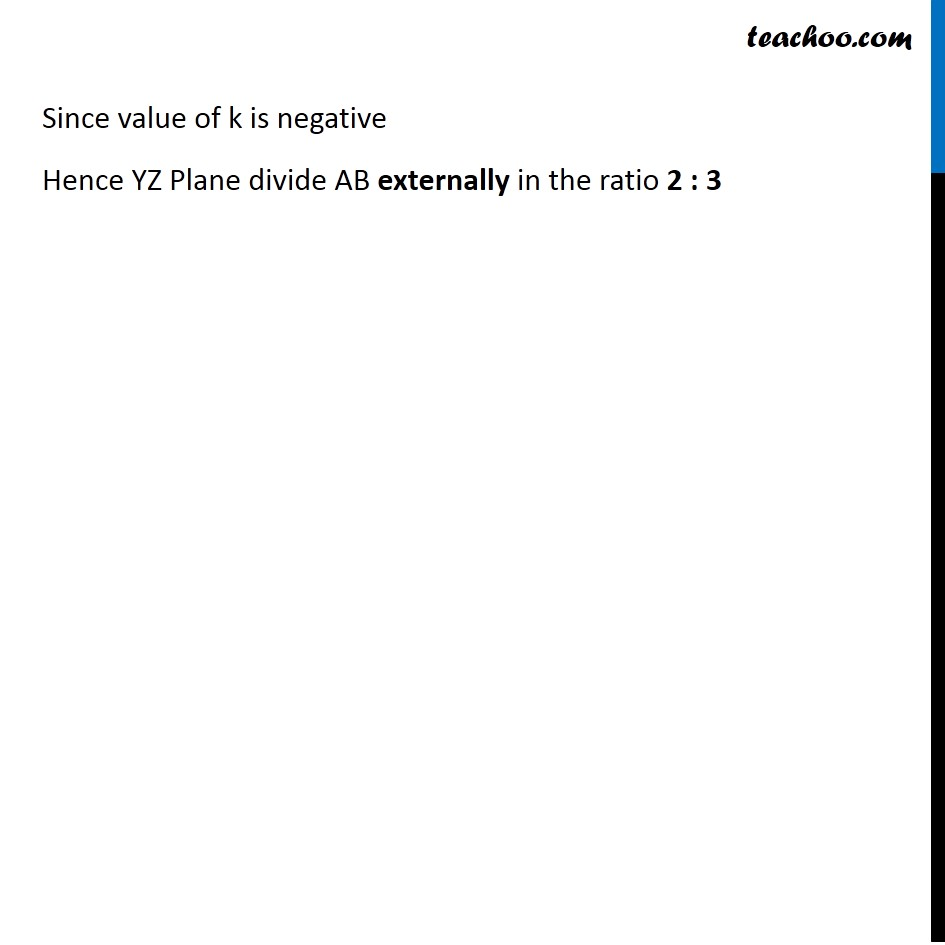Section - Plane dividing line segement

Chapter 12 Class 11 - Intro to Three Dimensional Geometry
Concept wiseIntroducing your new favourite teacher - Teachoo Black, at only ₹83 per month

### Transcript

Example 10 Find the ratio in which the line segment joining the points (4, 8, 10) and (6, 10, –8) is divided by the YZ-plane. Let AB be the line segment joining points A (4, 8, 10) & (6, 10, –8) Let YZ Plane divide line AB at P (x, y, z) in the ratio k : 1 Co-ordinate of P that divide line segment joining point A (x1, y1, z1) & B((x2, y2, z2) in the ratio m : n is = ((𝑚𝑥2 + 𝑛𝑥1)/(𝑚 + 𝑛), (𝑚𝑦2 + 𝑛𝑦1)/(𝑚 + 𝑛),(𝑚𝑧2 + 𝑛𝑧1)/(𝑚 + 𝑛)) Here, m = k , n = 1 x1 = 4, y1 = 8, z1 = 10 x2 = 6 , y2 = 10, z2 = –8 Co- ordinate of P P (x, y , z) = ((𝑘 (6) + 1 (4))/(𝑘 + 1), (𝑘(10) + 1(8))/(𝑘 + 1), (𝑘(−8) + 1(10))/(𝑘 + 1)) P (x, y , z) = ((6𝑘 + 4)/(k + 1),(10k + 8)/(k + 1),(−8𝑘 + 10)/(k + 1)) Since Point P (x, y, z) lies on the YZ plane its x – coordinate will be zero P (0, y , z) = ((6𝑘 + 4)/(k + 1),(10k + 8)/(k + 1),(−8𝑘 + 10)/(k + 1)) Comparing x – Coordinate 0 = (6𝑘 + 4)/(𝑘 + 1) (k + 1) (0) = 6k + 4 0 = 6k + 4 6k + 4 = 0 6k = – 4 k = (−4)/6 k = (−2)/3 𝑘/1 = (−2)/3 Thus, k : 1 = – 2 : 3 Since value of k is negative Hence YZ Plane divide AB externally in the ratio 2 : 3